Himanshi Singh — Published On March 16, 2021 and Last Modified On March 18th, 2021

## Introduction

Deep learning techniques are popularly used for unstructured data such as text data or image data. And before working on any type of data one should have a good understanding of it.

So in this article, we are going to discuss images and going to see how are they actually stored on a computer. We are going to cover two popular formats in which images are saved – Grayscale and RGB format.

Without any delay let’s begin!

Note: If you are more interested in learning the concept of Grayscale and RGB in an Audio-Visual format, We have this entire article explained in the video below. If not, you may continue reading.

## How B&W or Grayscale images are stored in a Computer?

Let’s take an example. Here we have taken a black and white image, also known as a Grayscale image.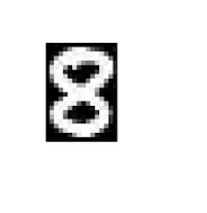This is the image of a number 8. Now, if we zoom in further and if you look closely you can see that the images getting distorted and you would see some small square boxes on this image.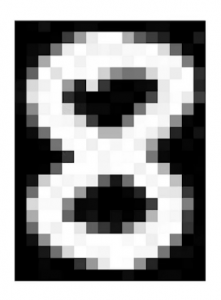These small boxes are called Pixels. We often use the term- the dimension of the image is X x Y. What does that actually mean? This means that the dimension of the image is simply the number of pixels across the height(x) and width(y) of the image. In this case, if you count, it would be 24 pixels across the height and 16 pixels across the width. Hence the dimension of this image will be 24 x 16. Although we see an image in this format the computer store image in the form of numbers-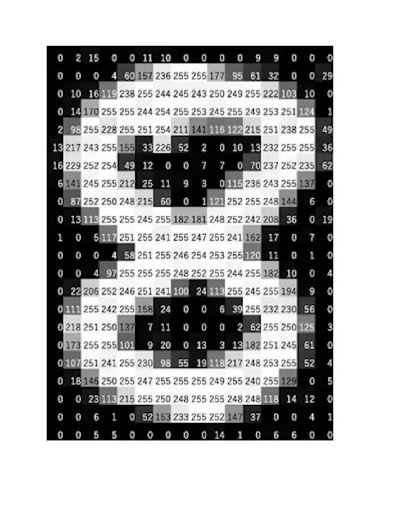Each of these pixels is denoted as the numerical value and these numbers are called Pixel Values. These pixel values denote the intensity of the pixels. For a grayscale or b&w image, we have pixel values ranging from 0 to 255.

The smaller numbers closer to zero represent the darker shade while the larger numbers closer to 255 represent the lighter or the white shade.

So every image in a computer is saved in this form where you have a matrix of numbers and this matrix is also known as a Channel-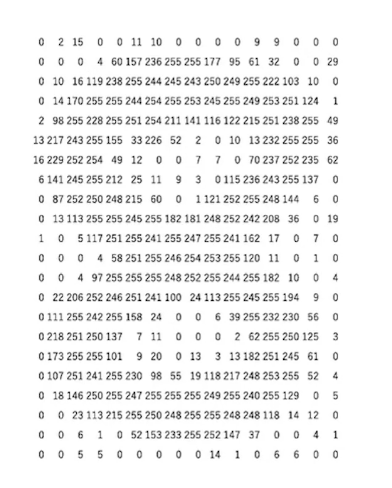Now can you guess the shape of this matrix? Well, it will be the same as the number of pixel values across the height and width of the image. In this case, the shape of the matrix would be 24 x 16

Now let’s quickly summarize the points that we’ve learned so far-

• Images are stored in the form of a matrix of numbers in a computer where these numbers are known as pixel values.
• These pixel values represent the intensity of each pixel.
• 0 represents black and 255 represents white.
• The matrix of numbers is known as the channel and in the case of a grayscale image, we have only one channel.

## How Colored images are stored on a computer?

Now that we have an idea about how are grayscale images stored in a computer, let’s look at an example for a colored image. So let’s take an example of a colored image, this is an image of a dog-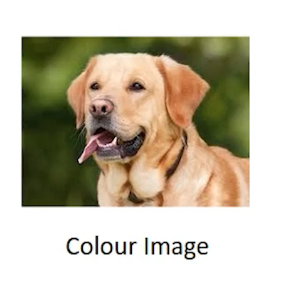This image is composed of many colors and almost all colors can be generated from the three primary colors- Red, Green, and Blue. We can say that each colored image is composed of these three colors or 3 channels- Red, Green, and Blue-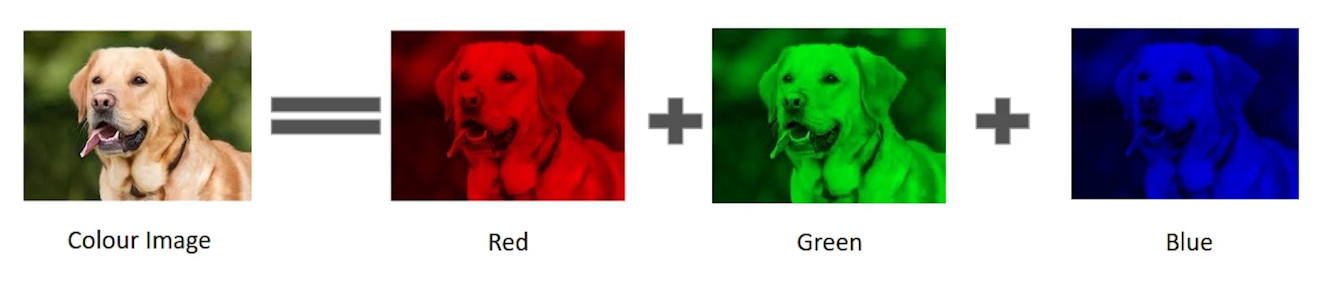This means that in a colored image the number of matrices or the number of channels will be more. In this particular example, we have 3 matrices- 1 matrix for red known as Red channel-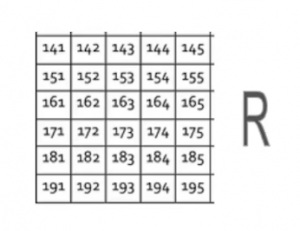another metrics for green known as the Green channel-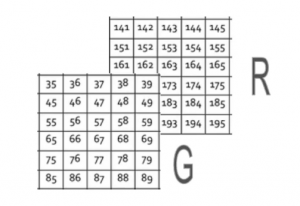and finally a matrix for the blue color also known as the Blue channel.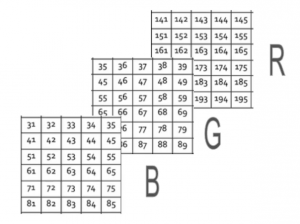Each of these metrics would again have values ranging from 0 to 255 where each of these numbers represents the intensity of the pixels or you can say that the shades of red, green, and blue. Finally, all of these channels or all of these matrices are superimposed so the shape of the image, when loaded in a computer, will be-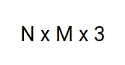where N is the number of pixels across the height, M would be the number of pixels across the width, and 3 is representing the number of channels, in this case, we have 3 channels R, G, and B. In our example, the shape of the colored image would be-     6 x 5 x 3 since we have 6 pixels across the height, 5 across the width and there are 3 channels present.

## End Notes

In this article, I covered the two most common image formats- Grayscale and RGB and how they’re stored in a computer. There are other formats of images as well which I can cover in the next article!

If you are looking to kick start your Data Science Journey and want every topic under one roof, your search stops here. Check out Analytics Vidhya’s Certified AI & ML BlackBelt Plus Program

If you have any queries regarding the concepts of Grayscale and RGB let me know in the comment section!

### About the Author###### Himanshi Singh

I am a data lover and I love to extract and understand the hidden patterns in the data. I want to learn and grow in the field of Machine Learning and Data Science.

## 2 thoughts on "How Images are stored in the computer?"###### Ganesh Chalamalasetti says:April 21, 2021 at 3:17 am
Dear Himanshi, Thanks for your article. I would like to share my knowledge as well regarding the Grayscale, B&W, and Colored images. As mentioned in your article, B&W and grayscale images can be called the same, I would rather consider that statement as wrong because B&W image pixels have values of either 0(black) or 255(white). Whereas grayscale image pixels can have values from 0 to 255 (shades of gray) but the three channels of the image will have the same pixel value (R=G=B). Let me know if you would like to share your inference on my comment. Thank you. Regards, Ganesh Reply###### Himanshi Singh says:April 22, 2021 at 11:44 am
Thanks a lot, Ganesh for adding this interesting information. It's always good to learn about new things. Reply